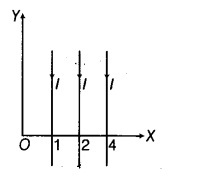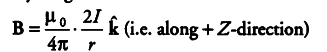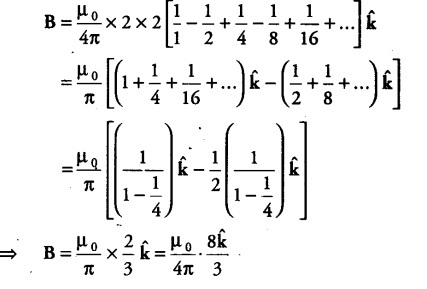# Find the magnetic field at the origin O

Equal currents I = 2 A are flowing through the infinitely long wires parallel to Y-axis located at x = + 1m, x = + 2m, x = + 4m and so on, but in opposite directions as shown in the figure. Find the magnetic field at the origin O.Magnetic field at O due to current through one infinitely long wire isSo, magnetic field at O due to all the wires is